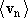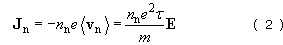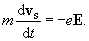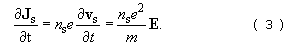Superconductivity

Start this free course now. Just create an account and sign in. Enrol and complete the course for a free statement of participation or digital badge if available.

Free course

# 3 Modelling properties of superconductors

## 3.1 A two-fluid model

As was mentioned earlier, a substantial dose of quantum mechanics would be required to provide a full explanation of the properties of superconductors. This would take us too far away from electromagnetism, and we shall therefore restrict our discussion to aspects that can be discussed using classical concepts of electromagnetism.

We shall model the free electrons within a superconductor as two fluids. According to this two-fluid model, one fluid consists of ‘normal’ electrons, number density n﻿n, and these behave in exactly the same way as the free electrons in a normal metal. They are accelerated by an electric field E, but are frequently scattered by impurities and defects in the ion lattice and by thermal vibrations of the lattice. The scattering limits the speed of the electrons, and they attain a mean drift velocity= −﻿e﻿﻿E / m, whereis the mean time between scattering events for the electrons and m is the electron mass. The current density Jn due to flow of these electrons isInterspersed with the normal electrons are what we shall call the superconducting electrons, or superelectrons, which form a fluid with number density ns. The superconducting electrons are not scattered by impurities, defects or thermal vibrations, so they are freely accelerated by an electric field. If the velocity of a superconducting electron is vs, then its equation of motion isCombining this with the expression for the current density, J﻿s = −﻿n﻿s﻿e﻿v﻿s, we find thatCompare this with Equation 2, which relates current density and electric field in a normal conductor. Scattering of the normal electrons leads to a constant current in a constant electric field, whereas the absence of scattering of the electrons in a superconductor means that the current density would increase steadily in a constant electric field. However, if we consider a constant current flowing in the superconductor, then ∂﻿J﻿s/∂﻿t = 0, so E = 0. Therefore the normal current density must be zero – all of the steady current in a superconductor is carried by the superconducting electrons. Of course, with no electric field within the superconductor, there will be no potential difference across it, and so it has zero resistance.

SMT359_1